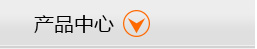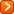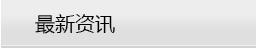# 康明斯柴油发电机组油耗一表可知

## 康明斯柴油发电机组油耗一表可知30kw康明斯柴油发电机组油耗量=6.3公斤(kg) =7.8升(L)

45kw康明斯柴油发电机组油耗量=9.45公斤(kg) =11.84升(L)

50kw康明斯柴油发电机组油耗量=10.5公斤(kg) =13.1升 (L)

75kw康明斯柴油发电机组油耗量=15.7公斤(kg) =19.7升 (L)

100kw康明斯柴油发电机组油耗量=21公斤(kg) =26.25升 (L)

150kw康明斯柴油发电机组油耗量=31.5公斤(kg) =39.4 升(L)

200kw康明斯柴油发电机组油耗量=40公斤(kg) =50升(L)
250kw康明斯柴油发电机组油耗量=52.5公斤(kg) =65.6升 (L)

300kw康明斯柴油发电机组油耗量=63公斤(kg) =78.75升 (L)

350kw康明斯柴油发电机组油耗量=73.5公斤(kg) =91.8升(L)
400kw康明斯柴油发电机组油耗量=84.00公斤(kg) =105.00 升(L)

450kw康明斯柴油发电机组油耗量=94.50公斤(kg) =118.00升 (L)

500kw康明斯柴油发电机组油耗量=105.00公斤(kg) =131.20升 (L)•康明斯
•玉柴
•潍柴
•帕金斯
•济柴
•上柴
•道依茨
•斗山
•重汽
•里卡多
•沃尔沃
•MTU
•10-30KW
•250KW
•450KW
•810-990KW
•35-50KW
•300KW
•500KW
•1000KW
•55-100KW
•350KW
•510-600KW
•1100-1500KW
•110-200KW
•400KW
•610-800KW
•1550-2000KW
•开架式机组
•柴油机组
•集装箱式机组
•静音式机组
•气体机组
•移动拖车式机组
•灯塔式机组
•船用机组
•移动应急电源车